# IB Physics Formulae You Need to Memorise### IB Physics Data Booklet: the formulae you MUST memorise to ace your exam!

The formulae below are not in the IB physics data booklet and you should memorise them!

BUT FIRST...

You should also make sure you have a copy of the IB physics data booklet and that you practise using it before your exams.

Now for the important part...

I'll walk through each topic and explain each equation that needs to be memorised.

#### Topic 1: Measurements and Uncertainties

One of the basic error propagation rules is not included. This is if you are multiplying a quantity by a constant factor with no associated error, then the absolute error is multiple by the constant too. However, the relative error omits the constant.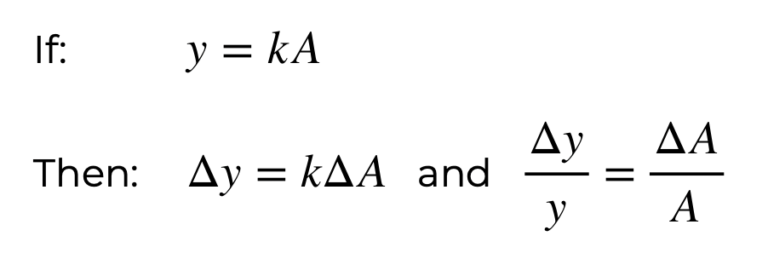All of the other formulae needed for manipulating errors are included in the data booklet.

#### Topic 2: Mechanics

For an object in freefall in a gravitational field, the total energy in the system is (assuming no air resistance or drag):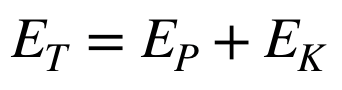Power in terms of work done: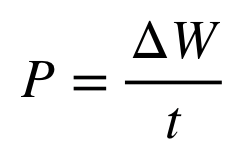#### Topic 3: Thermal Physics

The Stefan-Boltzmann constant (kb), the universal gas constant (R) and Avogadro’s number (NA) are all linked by: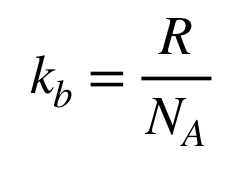#### Topic 4: Waves

Intensity of electromagnetic radiation at a distance r from a source with power, P.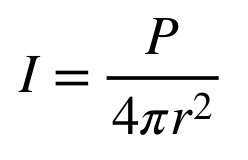#### Topic 5: Electricity and Magnetism

Another expression for the formula for internal resistance is: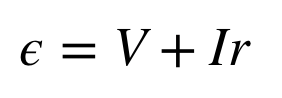#### Topic 6: Circular Motion and Gravitation

Average angular velocity is equal to the rate of change of angular displacement, θ, with respect to time, t.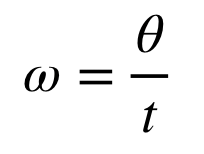#### Topic 7: Atomic, Nuclear & Particle Physics

Example of an alpha decay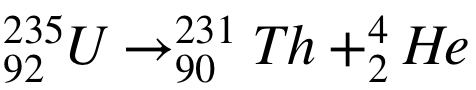Example of a beta decay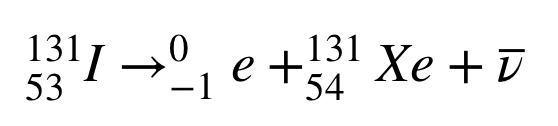#### Topic 8: Energy Production

If a wind turbine has a blade radius, r, then the area, A, swept our by the blades is πr² and the maximum theoretical kinetic energy arriving at the turbine every second (and hence the maximum theoretical power) is: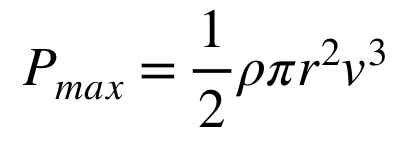This assumes that all the kinetic energy of the wind can be used, which  is not possible given that the wind will move out of the back of the turbine after moving the blades and will hence retain some of its kinetic energy.

#### Topic 9: Wave Phenomena

The defining equation of SHM is: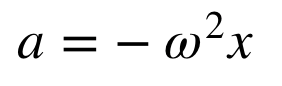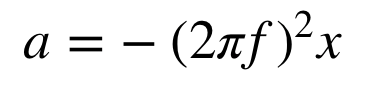#### Topic 10: Fields

You can find the relative permittivity (εr) in terms of the permittivity of the medium (ε) and vacuum (εo) is: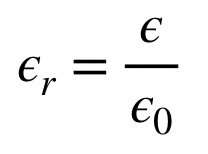The potential difference between two parallel plates, with voltage, V, and separation, d, is: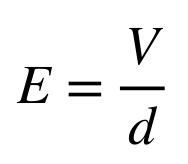#### Topic 11: Electromagnetic Induction

Maximum and minimum values for an emf in an ac generator is: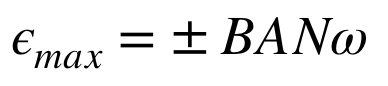#### Topic 12: Quantum and Nuclear Physics

de Broglie states that particles have wave-like properties and they are linked by the following equation: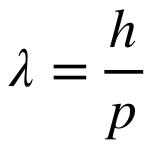Where λ is the wavelength, h is the planck’s constant and p is the momentum of the particle (p=mv)

If you memorise the formulae listed above NOT listed in the IB physics data booklet you will be well on the way to achieving a 7 in your IB physics exam! You might also want to:

Hope this helps!### FREE eBook: The ULTIMATE IB Physics Internal Assessment Guide